# Rates of Change and Behaviour of Graphs

Since functions represent how an output quantity varies with an input quantity, it is natural to ask about the rate at which the values of the function are changing.

For example, the function C(t) below gives the average cost, in dollars, of a gallon of gasoline t years after 2000.

 t 2 3 4 5 6 7 8 9 C(t) 1.47 1.69 1.94 2.3 2.51 2.64 3.01 2.14

If we were interested in how the gas prices had changed between 2002 and 2009, we could compute that the cost per gallon had increased from $1.47 to$2.14, an increase of $0.67. While this is interesting, it might be more useful to look at how much the price changed per year. You are probably noticing that the price didn’t change the same amount each year, so we would be finding the average rate of change over a specified amount of time. The gas price increased by$0.67 from 2002 to 2009, over 7 years, for an average of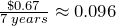dollars per year. On average, the price of gas increased by about 9.6 cents each year.

Rate of change: Describes how the output quantity changes in relation to the input quantity. The units on a rate of change are “output units per input units”.

Some other examples of rates of change include:

• A population of rats increases by 40 rats per week
• A barista earns $9 per hour (dollars per hour) • A farmer plants 60,000 onions per acre • A car can drive 27 miles per gallon • A population of grey whales decreases by 8 whales per year • The amount of money in your university account decreases by$4,000 per quarter

Average rate of change: The average rate of change between two input values is the total change of the function values (output values) divided by the change in the input values.

Average rate of change =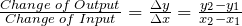Example 3.3.1

Using the cost-of-gas function from earlier, find the average rate of change between 2007 and 2009

From the table, in 2007 the cost of gas was $2.64. In 2009 the cost was$2.14.

The input (years) has changed by 2. The output has changed by $2.14 –$2.64 = -0.50. The average rate of change is then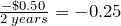dollars per year

Notice that in the last example the change of output was negative since the output value of the function had decreased. Correspondingly, the average rate of change is negative.

Example 3.3.2

Given the function g(t) shown here, find the average rate of change on the interval [0, 3].At t = 0, the graph shows g(0) = 1

At t = 3, the graph shows g(3) = 4

The output has changed by 3 while the input has changed by 3, giving an average rate of change of: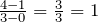Example 3.3.3

On a road trip, after picking up your friend who lives 16 km away, you decide to record your distance from home over time. Find your average speed over the first 6 hours.

 t (hours) 0 1 2 3 4 5 6 7 D(t) (km) 16 95 170 265 364 432 538 625

Here, your average speed is the average rate of change.

You traveled 538 km in 6 hours, for an average speed of: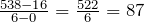km per hour.

We can more formally state the average rate of change calculation using function notation.

Average rate of change using function notation: Given a function f(x), the average rate of change on the interval [a, b] is

Average rate of change =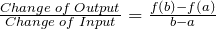Example 3.3.4

Compute the average rate of change ofon the interval [2, 4].

We can start by computing the function values at each endpoint of the interval: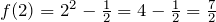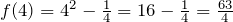Now computing the average rate of change:
Average rate of change =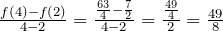Example 3.3.5

The magnetic force F, measured in Newtons, between two magnets is related to the distance between the magnets d, in centimetres, by the formula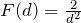. Find the average rate of change of force if the distance between the magnets is increased from 2 cm to 6 cm.

We are computing the average rate of change ofon the interval [2, 6]

 Average rate of change =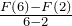Evaluating the function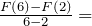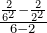Simplifying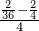Combining the numerator terms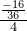Simplifying further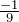Newtons per centimetre

This tells us the magnetic force decreases, on average, by 1/9 Newtons per centimetre over this interval.

# Graphical Behaviour of Functions

As part of exploring how functions change, it is interesting to explore the graphical behaviour of functions.

Increasing/decreasing:

A function is increasing on an interval if the function values increase as the inputs increase. More formally, a function is increasing if f(b) > f(a) for any two input values a and b in the interval with b > a. The average rate of change of an increasing function is positive.

A function is decreasing on an interval if the function values decrease as the inputs increase. More formally, a function is decreasing if f(b) < f(a) for any two input values a and b in the interval with b>a. The average rate of change of a decreasing function is negative.

Example 3.3.6

Given the function p(t) graphed here, on what intervals does the function appear to be increasing?The function appears to be increasing from t = 1 to t = 3, and from t = 4 on.

In interval notation, we would say the function appears to be increasing on the interval (1, 3) and the interval (4, ∞).

Notice in the last example that we used open intervals (intervals that don’t include the endpoints) since the function is neither increasing nor decreasing at t = 1, 3, or 4.

Local extrema: A point where a function changes from increasing to decreasing is called a local maximum.

A point where a function changes from decreasing to increasing is called a local minimum.

Together, local maxima and minima are called the local extrema, or local extreme values, of the function.

Example 3.3.7

Using the cost of gasoline function from the beginning of the section, find an interval on which the function appears to be decreasing. Estimate any local extrema using the table.

 t 2 3 4 5 6 7 8 9 C(t) 1.47 1.69 1.94 2.3 2.51 2.64 3.01 2.14

It appears that the cost of gas increased from t = 2 to t = 8. It appears the cost of gas decreased from t = 8 to t = 9, so the function appears to be decreasing on the interval (8, 9).

Since the function appears to change from increasing to decreasing at t = 8, there is local maximum at t = 8.

# Practice questions

1. Using the same cost-of-gas function, find the average rate of change between 2003 and 2008.

2. Find the average rate of change of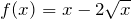on the interval [1, 9].

3. Find the average rate of change of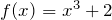on the interval [a, a + h].

4. Use the following graph of the function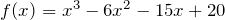to estimate the local extrema of the function. Determine the intervals on which the function is increasing and decreasing.5. Examine the graph below and answer the following questions.

a. What is the average rate of change over the time period of the graph?

b. How many local extrema occurred?

c. In what years did local maxima occur?